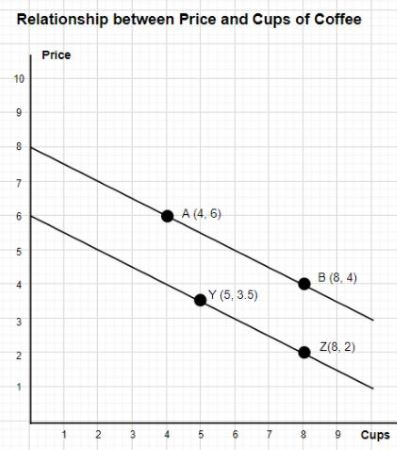# The slope of the line containing points Y and Z is ____. a. -0.5 b. -1 c. -2 d. -4

## Question:The slope of the line containing points Y and Z is _____.

a. -0.5

b. -1

c. -2

d. -4

## Slope:

The slope is used to measure the behavior of random variables. Any change in the value of random variables is analyzed by the slope. It represents the rate of change in the variable. The change can be negative, as well as positive.